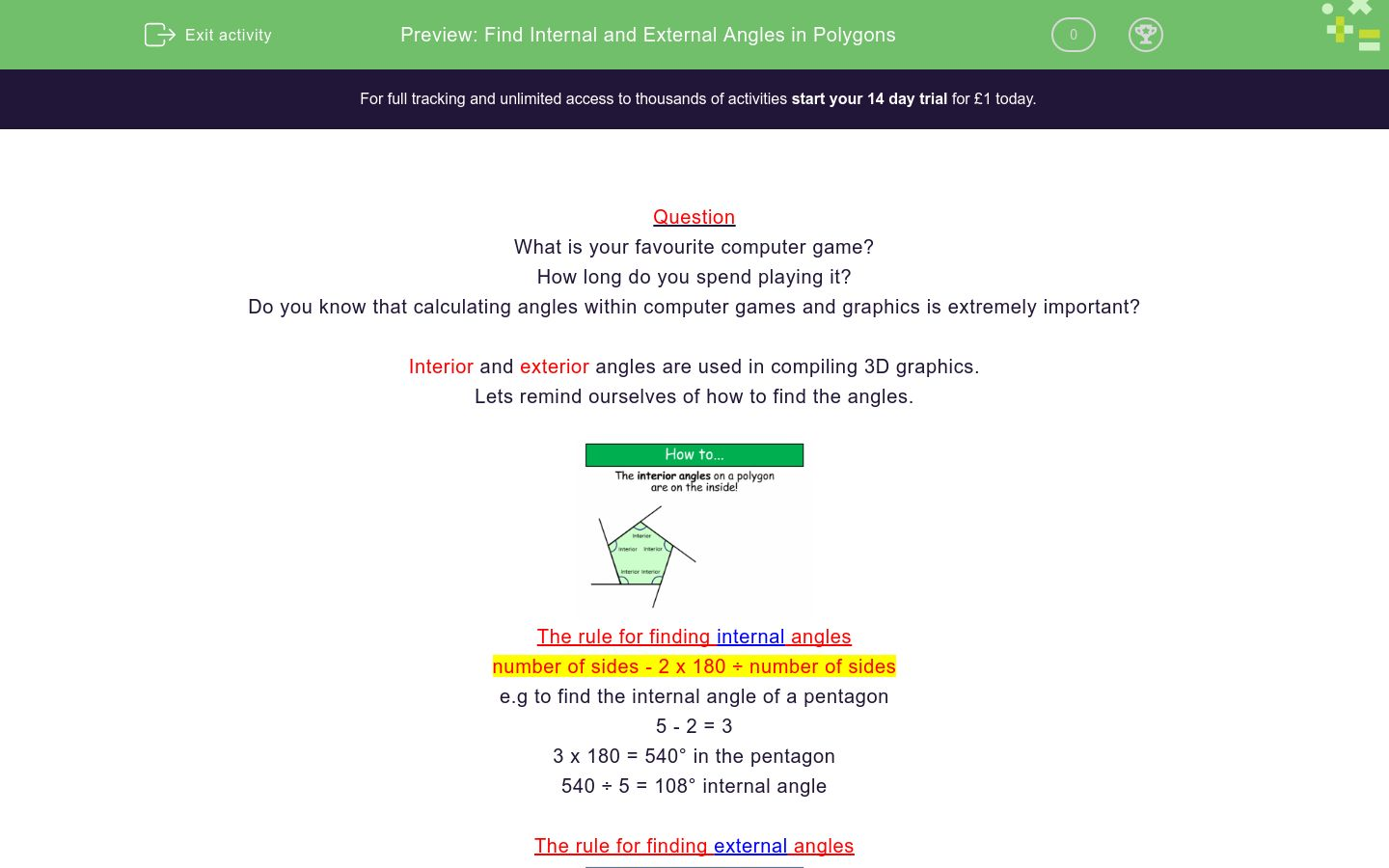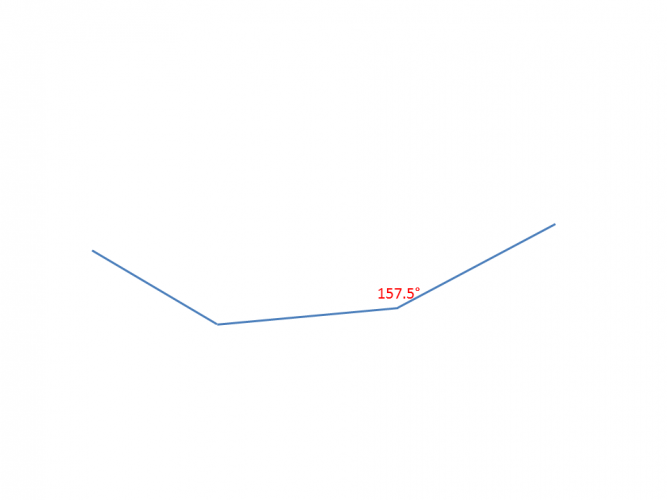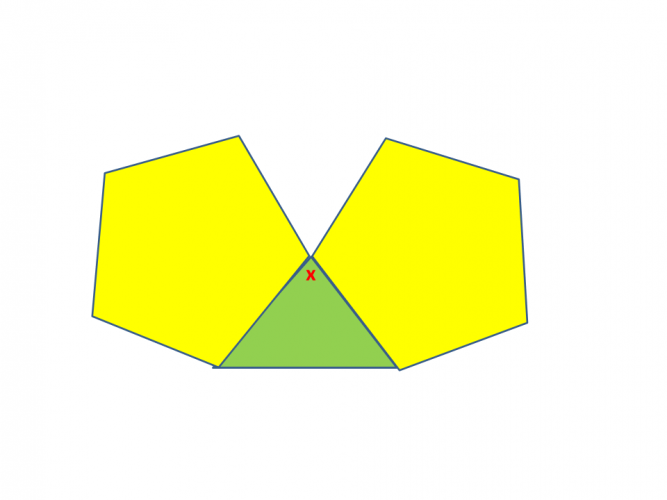# Find Internal and External Angles in Polygons

In this worksheet, students will find internal and external angles of regular polygons and solve simple problems.Key stage:  KS 4

GCSE Subjects:   Maths

GCSE Boards:   AQA, Eduqas, Pearson Edexcel, OCR

Curriculum topic:   Geometry and Measures, Basic Geometry

Curriculum subtopic:   Properties and Constructions, Angles

Difficulty level:### QUESTION 1 of 10

Question

What is your favourite computer game?

How long do you spend playing it?

Do you know that calculating angles within computer games and graphics is extremely important?

Interior and exterior angles are used in compiling 3D graphics.

Lets remind ourselves of how to find the angles.The rule for finding internal angles

number of sides - 2 x 180 ÷ number of sides

e.g to find the internal angle of a pentagon

5 - 2 = 3

3 x 180 = 540° in the pentagon

540 ÷ 5 = 108° internal angle

The rule for finding external anglesLet us look at the rule for internal and external angles.Example

Find the interior and exterior angle of this heptagon.Interior

7 - 2 = 5

5 x 180 = 900

900 ÷ 7 = 128.5°

Exterior

360 ÷ = 51.5°

Check

128.5° + 51.5° = 180°

Time to get off the computer games and get to work.140° 144° 135° 36° 40° 45° The exterior angle of an Octagon is The interior angle of an Octagon is140° 144° 135° 36° 40° 45° The exterior angle of an Octagon is The interior angle of an Octagon isThis diagram shows part of a regular polygon.

Each interior angle is 157.5°

a) What is the size of the exterior angle?

b) How many sides does the polygon have?

 140° 144° 135° 36° 40° 45° The exterior angle of an Octagon is The interior angle of an Octagon isSam here measured all the interior angles in a polygon.  He added them up to get 842°, but he had missed one angle.

a) What type of polygon did Sam measure?

b) What is the size of the missing angle?

 140° 144° 135° 36° 40° 45° The exterior angle of an Octagon is The interior angle of an Octagon isTwo regular pentagons are put together.

What is the value of x?A regular pentagon and hexagon are placed together.

What is the value of x?What are the interior and exterior angles of a 15 sided regular polygon?The value of x is 60° 68° 72°Calculate the value of x.This star has 10 sides that are equal in length.

The reflex angle is 200°

• Question 1140° 144° 135° 36° 40° 45° The exterior angle of an Octagon is The interior angle of an Octagon is
EDDIE SAYS
180 and 360 are useful numbers to remember in maths. They help us in all sorts of areas. We just need to learn the different rules they offer us. Exterior angle 360 ÷ 8 = 45° Interior angle 8 - 2 = 6 6 x 180 = 1080 1080 ÷ 8 = 135°
• Question 2EDDIE SAYS
Did you know that a 20 sided shape is called an isocagon? You can wow your friends with that one at the next quiz. Interior angle 18 x 180 = 3240 ÷ 20 = 162 Exterior angle 360 ÷ 20 = 18 162 + 18 = 180°
• Question 3This diagram shows part of a regular polygon.

Each interior angle is 157.5°

a) What is the size of the exterior angle?

b) How many sides does the polygon have?

EDDIE SAYS
This time you have to use the fact that interior and exterior angles add up to 180° 180 - 157.5° = 22.5° To find the number of sides 360 ÷ 22.5 = 16 Its a hexadecagon in case you are wondering for the maths quiz.
• Question 4Sam here measured all the interior angles in a polygon.  He added them up to get 842°, but he had missed one angle.

a) What type of polygon did Sam measure?

b) What is the size of the missing angle?

EDDIE SAYS
Here it is useful to know the sum of the interior angles. If you don't, its not a problem, just start at 180 for a triangle, add 180 to get a quadrilateral, add 180 for a pentagon and so on. Either way Sam has measured the polygon just short of 900° which is a heptagon. To find the missing angle 900 - 842 = 58°
• Question 5Two regular pentagons are put together.

What is the value of x?

144
EDDIE SAYS
Using our calculations to find the interior angle of a pentagon we know that the interior angle of the pentagon is 108° Around x there are two lots of 108° We have to draw on the knowledge that angles around a point add up to 360° SO 108 + 108 = 216° 360 - 216 = 144°
• Question 6A regular pentagon and hexagon are placed together.

What is the value of x?

132
EDDIE SAYS
Hopefully you are getting used to applying the formulas now. We need to find the interior angles of the pentagon and hexagon first. 3 x 180 = 540 ÷ 5 = 108° 4 x 180 = 720 ÷ 6 = 120° 120 + 108 = 228° 360 - 228 = 132°
• Question 7What are the interior and exterior angles of a 15 sided regular polygon?

EDDIE SAYS
The interior angle is 13 x 180 (don't forget to take two off the number of sides) ÷ 15 = 156° Interior and exterior angles add up to 180° 180 - 156 = 24°
• Question 8The value of x is 60° 68° 72°
EDDIE SAYS
We are looking at internal angles only here to start with. Find the angles of the inside of the pentagon. 3 x 180 = 540 ÷ 5 = 108° angles 108 are either side of the x gives us 216° Angles around a point add up to 360° 360 - 216 = 144° We have know that opposite angles are equal therefore 144 ÷ 2 = 72°
• Question 9Calculate the value of x.

54
EDDIE SAYS
Calculate the interior angle of the pentagon which is 108° Each interior angle in the square is 90° To find x 108 + 108 + 90 = 306° 360 - 306° = 54° this gives us the external angle.
• Question 10This star has 10 sides that are equal in length.

The reflex angle is 200°

88
EDDIE SAYS
Oh my there is quite a bit to do here. First find the sum of the angles in the 10 sided shape. 8 x 180 = 1440° Next find the sum of the reflex angles. 200 x 5 = 1000 The remaining 440 degrees are shared between the 5 acute angles. 440°÷ 5 = 88° What a star you are.
---- OR ----

Sign up for a £1 trial so you can track and measure your child's progress on this activity.

### What is EdPlace?

We're your National Curriculum aligned online education content provider helping each child succeed in English, maths and science from year 1 to GCSE. With an EdPlace account you’ll be able to track and measure progress, helping each child achieve their best. We build confidence and attainment by personalising each child’s learning at a level that suits them.

Get started